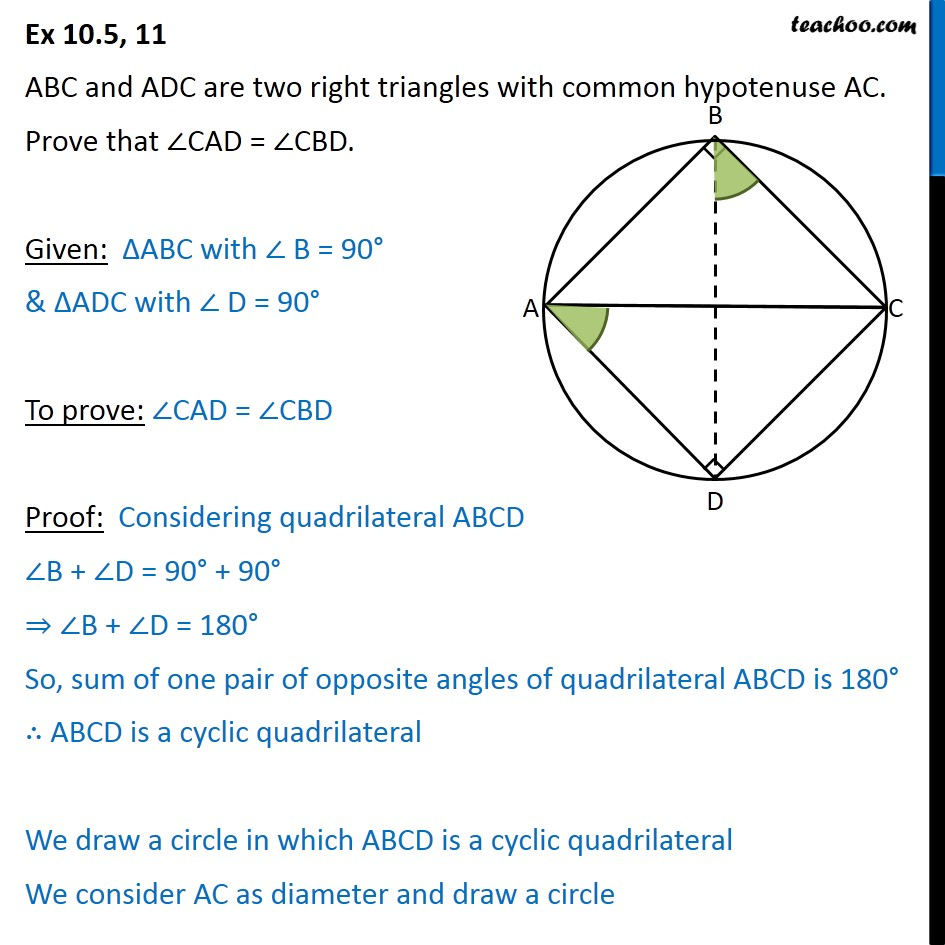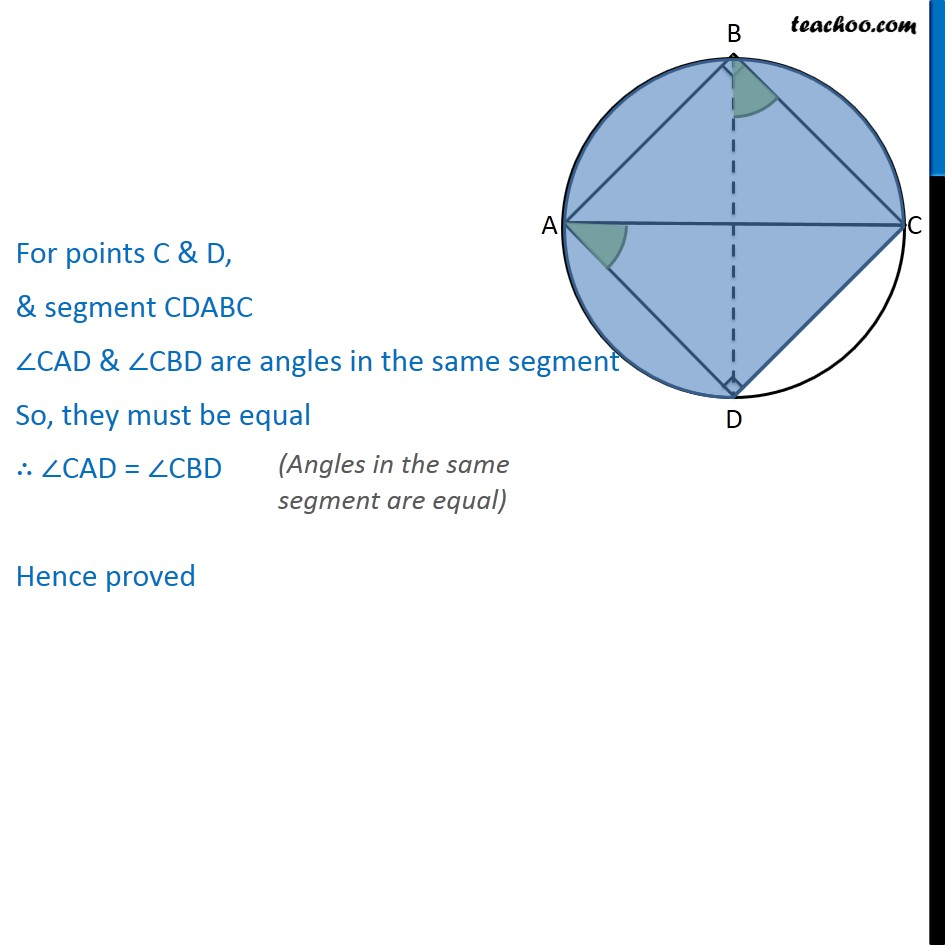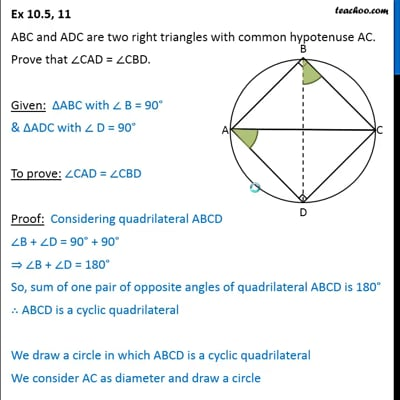Ex 10.5

Chapter 10 Class 9 Circles
Serial order wiseThis video is only available for Teachoo black users

Solve all your doubts with Teachoo Black (new monthly pack available now!)

### Transcript

Ex 10.5, 11 ABC and ADC are two right triangles with common hypotenuse AC. Prove that ∠CAD = ∠CBD. Given: ∆ABC with ∠ B = 90° & ∆ADC with ∠ D = 90° To prove: ∠CAD = ∠CBD Proof: Considering quadrilateral ABCD ∠B + ∠D = 90° + 90° ⇒ ∠B + ∠D = 180° So, sum of one pair of opposite angles of quadrilateral ABCD is 180° ∴ ABCD is a cyclic quadrilateral We draw a circle in which ABCD is a cyclic quadrilateral We consider AC as diameter and draw a circle For points C & D, & segment CDABC ∠CAD & ∠CBD are angles in the same segment So, they must be equal ∴ ∠CAD = ∠CBD Hence proved